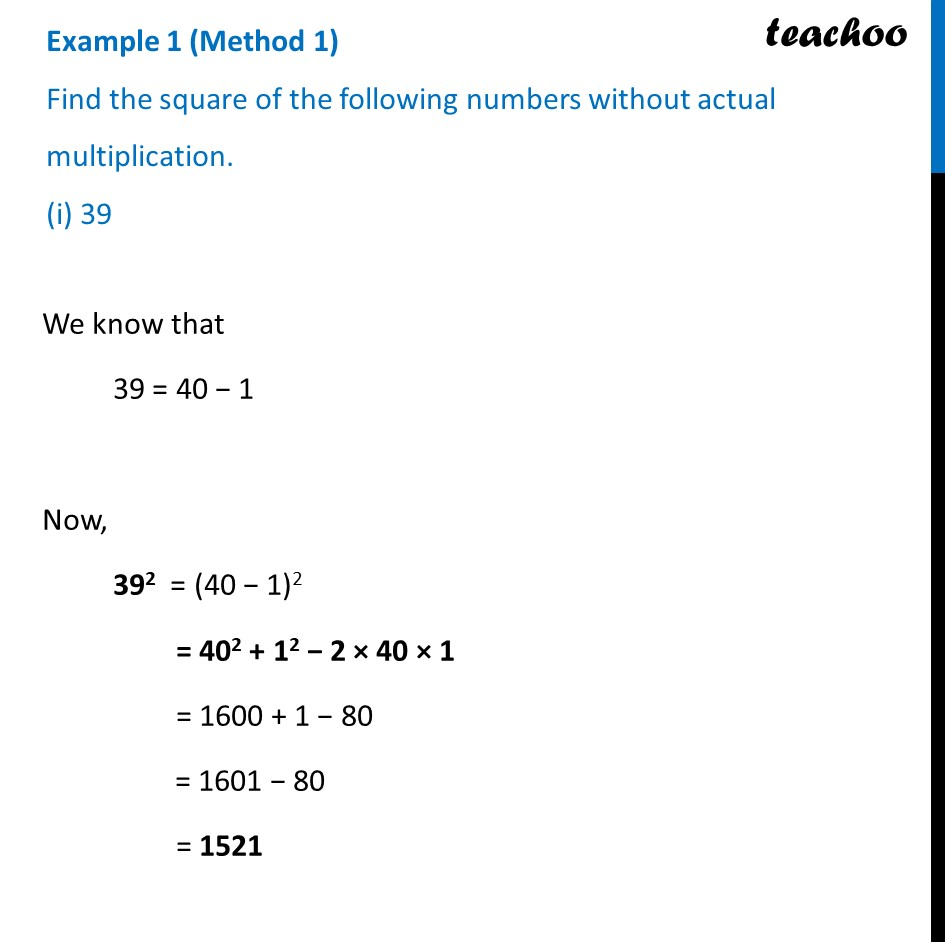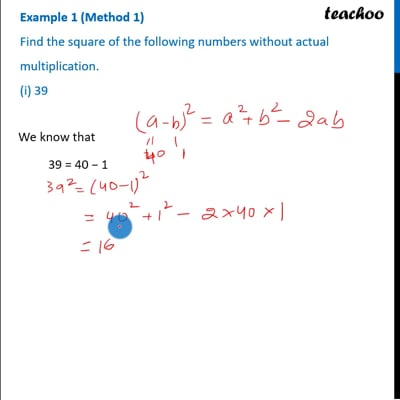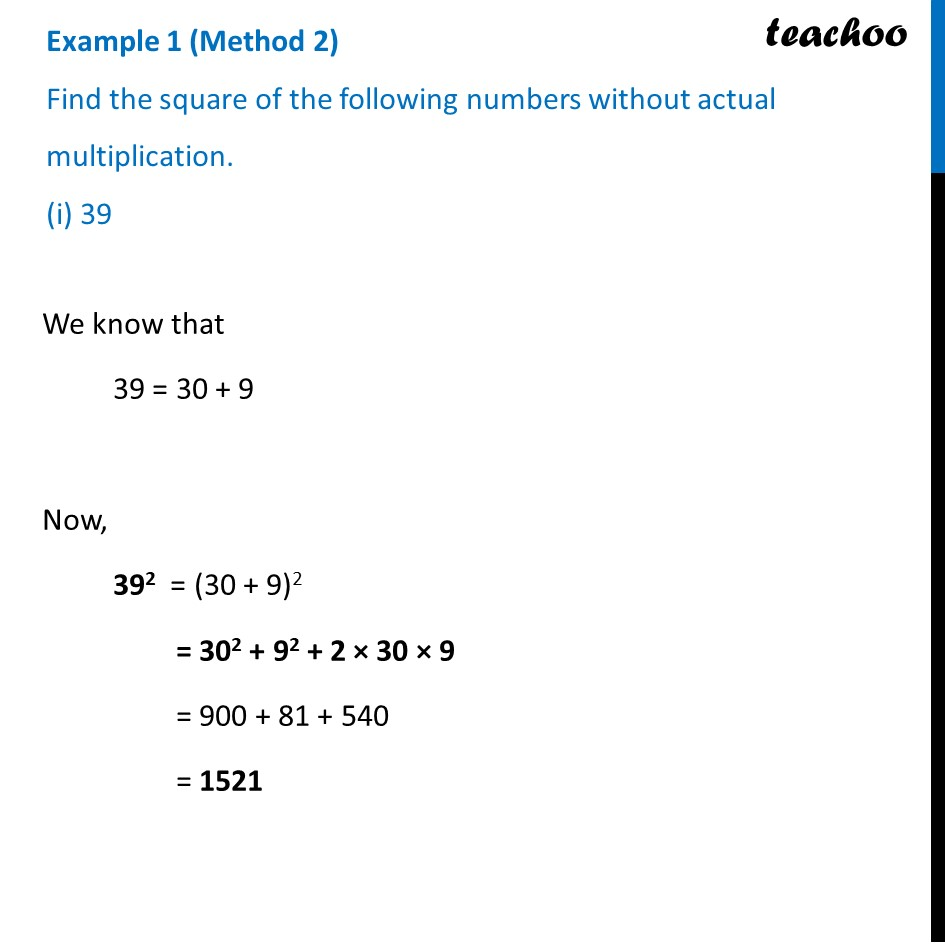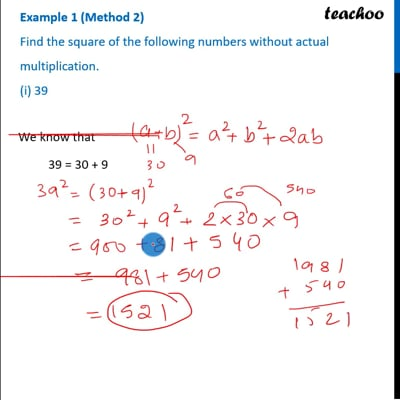Finding square of large numbers

Chapter 6 Class 8 Squares and Square Roots
Concept wiseThis video is only available for Teachoo black usersThis video is only available for Teachoo black users

Introducing your new favourite teacher - Teachoo Black, at only ₹83 per month

### Transcript

Example 1 (Method 1) Find the square of the following numbers without actual multiplication. (i) 39 We know that 39 = 40 − 1 Now, 392 = (40 − 1)2 = 402 + 12 − 2 × 40 × 1 = 1600 + 1 − 80 = 1601 − 80 = 1521 Example 1 (Method 2) Find the square of the following numbers without actual multiplication. (i) 39 We know that 39 = 30 + 9 Now, 392 = (30 + 9)2 = 302 + 92 + 2 × 30 × 9 = 900 + 81 + 540 = 1521